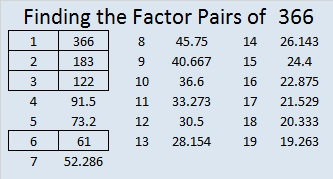# 366 and a Quote from Euclid

The laws of nature are but the mathematical thoughts of God.  ~Euclid

Euclid was a Greek mathematician who was born about 300 BC. I realize when he wrote these words (in ancient Greek), they didn’t mean the exact same thing to him that they mean to me. Nevertheless, I like the quote very much, and find infinite beauty in its message. The laws of nature are but the mathematical thoughts of God.  ~Euclid

Since writing this post, I have found some other lovely representations of this quote on twitter:

It is well worth pushing the button to see this next gif in action:

Here are some mathematical thoughts about the number 366:

• 366 is a composite number.
• Prime factorization: 366 = 2 x 3 x 61
• The exponents in the prime factorization are 1, 1, and 1. Adding one to each and multiplying we get (1 + 1)(1 + 1)(1 + 1) = 2 x 2 x 2 = 8. Therefore 366 has exactly 8 factors.
• Factors of 366: 1, 2, 3, 6, 61, 122, 183, 366
• Factor pairs: 366 = 1 x 366, 2 x 183, 3 x 122, or 6 x 61
• 366 has no square factors that allow its square root to be simplified. √366 ≈ 19.13166² + 360² = 366² How do I know? Because 11² + 60² = 61² so (6·11)² + (6·60)² = (6·61)².

366 is a palindrome in a couple of bases:

• 303 in BASE 11 because 3(11²) + 0(11¹) + 3(11º) = 366
• 222 in BASE 13 because 2(13²) + 2(13¹) + 2(13º) = 366

These are just a few of the things known by the Greatest Mathematician of All! He completely understands EVERYTHING from infinitesimal to infinity. By His grace I understand a tiny bit of what He knows.

This site uses Akismet to reduce spam. Learn how your comment data is processed.